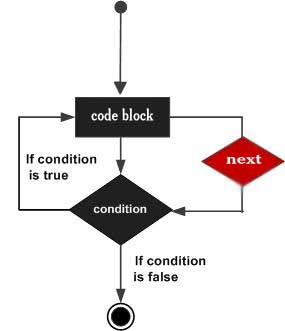# Perl next Statement

The Perl next statement starts the next iteration of the loop. You can provide a LABEL with next statement where LABEL is the label for a loop. A next statement can be used inside a nested loop where it will be applicable to the nearest loop if a LABEL is not specified.

If there is a continue block on the loop, it is always executed just before the condition is about to be evaluated. You will see the continue statement in separate chapter.

## Syntax

The syntax of a next statement in Perl is −

```next [ LABEL ];
```

A LABEL inside the square braces indicates that LABEL is optional and if a LABEL is not specified, then next statement will jump the control to the next iteration of the nearest loop.

## Flow Diagram## Example

```#!/usr/local/bin/perl

\$a = 10;
while( \$a < 20 ) {
if( \$a == 15) {
# skip the iteration.
\$a = \$a + 1;
next;
}
print "value of a: \$a\n";
\$a = \$a + 1;
}
```

When the above code is executed, it produces the following result −

```value of a: 10
value of a: 11
value of a: 12
value of a: 13
value of a: 14
value of a: 16
value of a: 17
value of a: 18
value of a: 19
```

Let's take one example where we are going to use a LABEL along with next statement −

```#!/usr/local/bin/perl

\$a = 0;
OUTER: while( \$a < 4 ) {
\$b = 0;
print "value of a: \$a\n";
INNER:while ( \$b < 4) {
if( \$a == 2) {
\$a = \$a + 1;
next OUTER;
}
\$b = \$b + 1;
print "Value of b : \$b\n";
}
print "\n";
\$a = \$a + 1;
}
```

When the above code is executed, it produces the following result −

```value of a : 0
Value of b : 1
Value of b : 2
Value of b : 3
Value of b : 4

value of a : 1
Value of b : 1
Value of b : 2
Value of b : 3
Value of b : 4

value of a : 2
value of a : 3
Value of b : 1
Value of b : 2
Value of b : 3
Value of b : 4
```
perl_loops.htm